## Input PWM is zero for SDK

cancel
Showing results for
Did you mean:
SOLVED

## Input PWM is zero for SDK

1,033 ViewsContributor II

Hello Community

We want to detect the Crash signal input and configure it according to the example, but there is no return value of IC_GETMEASUREMENT (), please help us to check whether we made a mistake in the configuration.

Chip version：S32K148 -> 144

PIN: PTD18

Config: FTM5_CH7

for example: ic_pal_s32k148

MCU detects CRASH signal input,

uint16_t inputCaptureMeas = 0U;
uint16_t inputMeas = 0U;
uint32_t frequency;
uint8_t channel = ic_pal1_ChnConfig->hwChannelId;

IC_Init(&ic_pal1_instance, &ic_pal1_InitConfig);

while(1)
{
frequency = FTM_DRV_GetFrequency(ic_pal1_instance.instIdx);
inputCaptureMeas = IC_GetMeasurement(&ic_pal1_instance, channel);
inputMeas = frequency / (inputCaptureMeas);

JPCC_DEBUG(JPCC_LOG_LEVEL_WARNING, "[ SensorApp] 6 PWM uFrequencyHZ=[%lu],         frequency=[%lu], inputCaptureMeas=[%u], inputMeas=[%lu]\r\n",
flexTimer_pwm1_PwmConfig.uFrequencyHZ, frequency, inputCaptureMeas, inputMeas);

OS_sleep(300);
};

1 Solution
848 ViewsNXP TechSupport

Hi,

Looking at the screenshots you posted, I see the FTM frequency is set to 256Hz.

The resolution is therefore 1/256 = 3.9ms

3.9ms * 28 = 109.2ms which is 91% of 120ms.

If you need to have a higher resolution, you need to set a higher FTM frequency.

Regards,

Daniel

9 Replies
931 ViewsContributor II

The signal input frequency we use is as shown in "crah.jpg"，I don't know how to calculate the duty cycle according to them. Could you please give me a calculation method or formula?

964 ViewsContributor II

Configuration changes

PIN: PTC10

Config: FTM3_CH4

The current configuration has SRS input.

We would like to know how to calculate the duty cycle. Could you provide a specific calculation formula?

6148 = IC_GetMeasurement(&ic_pal1_instance, channel);

duty = 40%.

949 ViewsNXP TechSupport

Hi,

The IC_GetMeasurement() function returns the duty-cycle value in FTM3 ticks (24MHz).

If you know the period of the input signal, you can easily calculate the duty-cycle in %.

Regards,

Daniel

929 ViewsContributor II

Our input signal (Type)

T = 120ms.

Hz = 0.0083333333333KHz

How do we use the data we have to calculate the duty cycle?Could you give me an example? Thank you

912 ViewsNXP TechSupport

Hi,

The IC_GetMeasurement() function returns number of FTM ticks measured while the input signal is ON.

One FTM tick is one period of the FTM function clock.

Instead of the PAL driver, you can use the FTM_IC driver.

Example: ftm_signal_measurement_s32k148

With this configuration:The driver can measure input signal: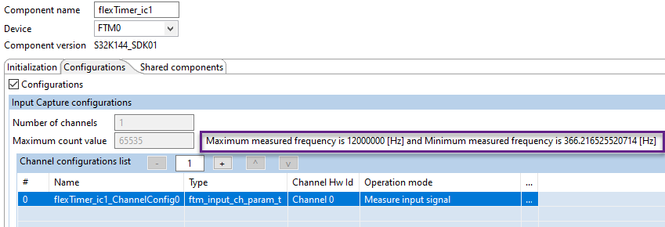If you need to measure signal of frequency 8.33Hz, you need to select a slower FTM clock.

For example,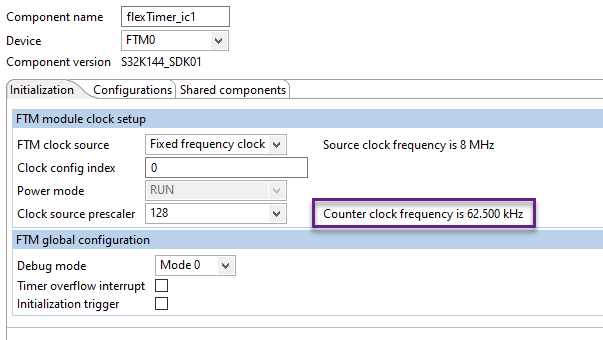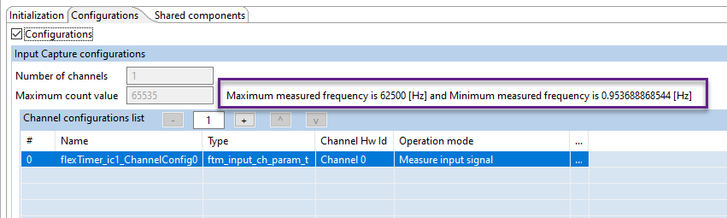In this case, 1 FTM clock cycle = 1 FTM tick = 1/62500 = 16us is resolution of the measurement.

You could use faster FTM clock frequency but you would need to count the FTM overflows and add it to the time captured between the two edges.

Regards,

Daniel

858 ViewsContributor II

Our input signal (Type)

T = 120ms.

Hz = 0.0083333333333KHz

Duty = 90%

FTM_DRV_GetInputCaptureMeasurement(INST_FLEXTIMER_IC1, 4U)  = 28;

1 FTM clock cycle = 1 FTM tick = 1/62500 = 16us is resolution of the measurement.

Duty cycle calculation formula:  (28 * 16us) / 120ms = 90%

Is that the calculation?

Could you specify the duty cycle calculation formula with the above parameters.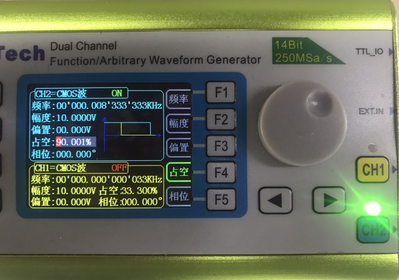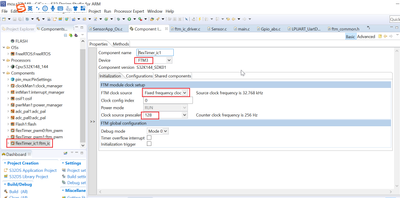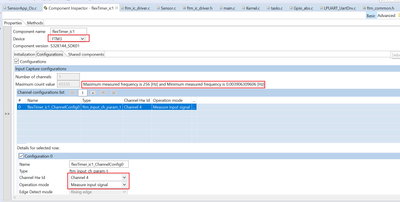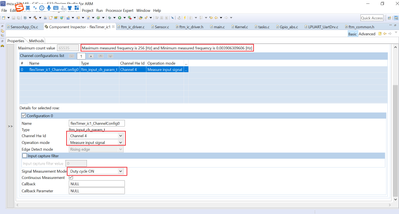849 ViewsNXP TechSupport

Hi,

Looking at the screenshots you posted, I see the FTM frequency is set to 256Hz.

The resolution is therefore 1/256 = 3.9ms

3.9ms * 28 = 109.2ms which is 91% of 120ms.

If you need to have a higher resolution, you need to set a higher FTM frequency.

Regards,

Daniel

1,003 ViewsNXP TechSupport

Hello,

Could you please attach the project?

The issue could be in the PIN driver, for example.

Can you scope the signal on PTD18?

Thank you,

BR, Daniel

1,026 ViewsContributor II

Analog CRASH signal